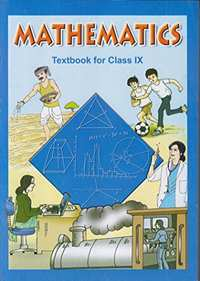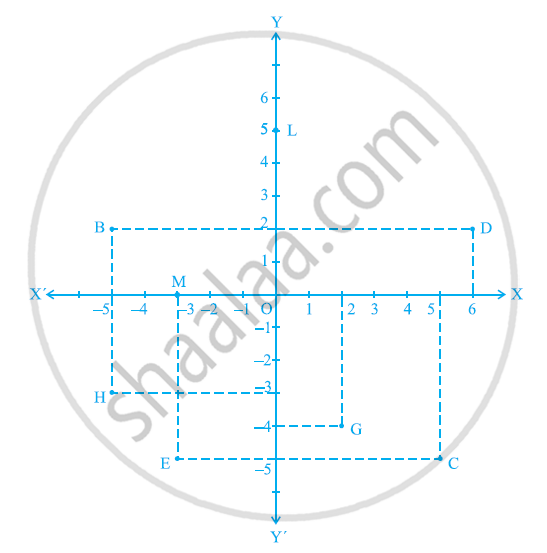Share

# NCERT solutions for Class 9 Mathematics Textbook chapter 3 - Coordinate Geometry [Latest edition]

Course
Textbook page

#### Chapters## Chapter 3: Coordinate Geometry

Ex. 3.1Ex. 3.2Ex. 3.3

#### NCERT solutions for Class 9 Mathematics Textbook Chapter 3 Coordinate Geometry Exercise 3.1 [Page 53]

Ex. 3.1 | Q 1 | Page 53

How will you describe the position of a table lamp on your study table to another person?

Ex. 3.1 | Q 2 | Page 53

(Street Plan) : A city has two main roads which cross each other at the centre of the city. These two roads are along the North-South direction and East-West direction.

All the other streets of the city run parallel to these roads and are 200 m apart. There are 5 streets in each direction. Using 1cm = 200 m, draw a model of the city on your notebook. Represent the roads/streets by single lines.

There are many cross- streets in your model. A particular cross-street is made by two streets, one running in the North - South direction and another in the East - West direction. Each cross street is referred to in the following manner : If the 2nd street running in the North - South direction and 5th in the East - West direction meet at some crossing, then we will call this cross-street (2, 5). Using this convention, find:-

(i) how many cross - streets can be referred to as (4, 3).

(ii) how many cross - streets can be referred to as (3, 4).

#### NCERT solutions for Class 9 Mathematics Textbook Chapter 3 Coordinate Geometry Exercise 3.2 [Page 60]

Ex. 3.2 | Q 1.1 | Page 60

Write the answer of the following question:-

What is the name of horizontal and the vertical lines drawn to determine the position of any point in the Cartesian plane?

Ex. 3.2 | Q 1.2 | Page 60

Write the answer of each of the following questions:-

What is the name of each part of the plane formed by these two lines?

Ex. 3.2 | Q 1.3 | Page 60

Write the answer of each of the following questions:-

Write the name of the point where these two lines intersect.

Ex. 3.2 | Q 2 | Page 60

See the given figure, and write the following:-

(i) The coordinates of B.

(ii) The coordinates of C.

(iii) The point identified by the coordinates (–3, –5).

(iv) The point identified by the coordinates (2, – 4).

(v) The abscissa of the point D.

(vi) The ordinate of the point H.

(vii) The coordinates of the point L.

(viii) The coordinates of the point M.#### NCERT solutions for Class 9 Mathematics Textbook Chapter 3 Coordinate Geometry Exercise 3.3 [Page 65]

Ex. 3.3 | Q 1 | Page 65

In which quadrant or on which axis do each of the points (– 2, 4), (3, – 1), (– 1, 0), (1, 2) and (– 3, – 5) lie? Verify your answer by locating them on the Cartesian plane.

Ex. 3.3 | Q 2 | Page 65

Plot the points (x, y) given in the following table on the plane, choosing suitable units of distance on the axes.

 x -2 -1 0 1 3 y 8 7 -1.25 3 -1

## Chapter 3: Coordinate Geometry

Ex. 3.1Ex. 3.2Ex. 3.3## NCERT solutions for Class 9 Mathematics Textbook chapter 3 - Coordinate Geometry

NCERT solutions for Class 9 Mathematics Textbook chapter 3 (Coordinate Geometry) include all questions with solution and detail explanation. This will clear students doubts about any question and improve application skills while preparing for board exams. The detailed, step-by-step solutions will help you understand the concepts better and clear your confusions, if any. Shaalaa.com has the CBSE Class 9 Mathematics Textbook solutions in a manner that help students grasp basic concepts better and faster.

Further, we at Shaalaa.com provide such solutions so that students can prepare for written exams. NCERT textbook solutions can be a core help for self-study and acts as a perfect self-help guidance for students.

Concepts covered in Class 9 Mathematics Textbook chapter 3 Coordinate Geometry are Introduction of Coordinate Geometry, Cartesian System, Plotting a Point in the Plane If Its Coordinates Are Given.

Using NCERT Class 9 solutions Coordinate Geometry exercise by students are an easy way to prepare for the exams, as they involve solutions arranged chapter-wise also page wise. The questions involved in NCERT Solutions are important questions that can be asked in the final exam. Maximum students of CBSE Class 9 prefer NCERT Textbook Solutions to score more in exam.

Get the free view of chapter 3 Coordinate Geometry Class 9 extra questions for Class 9 Mathematics Textbook and can use Shaalaa.com to keep it handy for your exam preparation

S